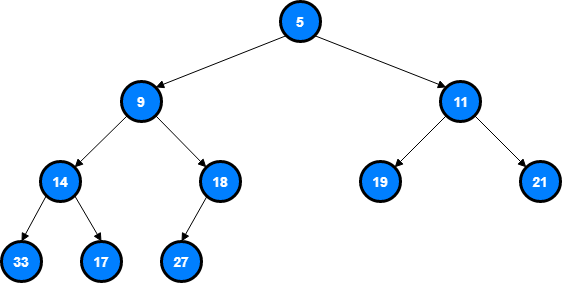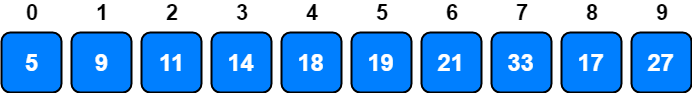# Heaps

The word heap is used in a couple of different context in Computer Science. A heap is sometimes refers to an area in the memory which is used for dynamic memory allocation. Another meaning, and the topic of this article, is a data structure which is very useful and important.

Famous search algorithms like Dijkstra’s algorithm1 and some reinforcement learning techniques like Hidden Markov Model (HMM)2 which is especially known for its application in pattern recognition (speech, handwriting, gesture recognition) uses the heap.

## What is a Heap?

A heap is a special Tree-based data structure in which the tree is a complete Binary Tree in which each level has all of its nodes. The exception to this is the bottom level of the tree, which we fill in from left to right.It is called;

• Min Heap, if each parent node is less than or equal to its child node
• Max Heap, if each parent node is greater than or equal to its child node

The heap above is called a Min Heap since, each value of nodes is less than or equal to the value of child nodes.

## Representation

We can represent a heap as an array like this;• Root node, i = 0 is the first item of the array
• A parent node, parent(i) = (i-1) / 2
• A left child node, left(i) = 2i + 1
• A right child node, right(i) = 2i + 2

For example,

• Parent node of 33 is, parent(7) = (7-1) / 2 = 3 so, i = 3 is 14
• Left child node of 11 is, left(2) = (2 x 2) + 1 = 5 so, i = 5 is 19
• Right child node of 14 is, right(3) = (2 x 3) + 2 = 8 so, i = 8 is 17

## Implementation

We need to implement two different methods in order to build a heap from an array. These methods are `min_heapify()` to make some node and its descendant nodes meet the min heap property and `build_min_heap()` to produce a heap from an arbitrary array.

We can build a min heap by applying `min_heapify()` repeatedly.

### `min_heapify()` and `build_min_heap()`

``````def get_left_child(i):
return 2 * i + 1

def get_right_child(i):
return 2 * i + 2

def min_heapify(arr, i):
left = get_left_child(i)
right = get_right_child(i)
smallest = i

if left < len(arr) and arr[i] > arr[left]:
smallest = left
if right < len(arr) and arr[smallest] > arr[right]:
smallest = right
if smallest != i:
arr[i], arr[smallest] = arr[smallest], arr[i]
min_heapify(arr, smallest)
``````

and now we can repeatedly call `min_heapify()` function in order to build a Min Heap.

``````def build_min_heap(arr):
for i in reversed(range(len(arr)//2)):
min_heapify(arr, i)
``````

### `max_heapify()` and `build_max_heap()`

``````def max_heapify(arr, i):
left = get_left_child(i)
right = get_right_child(i)
largest = i

if left < len(arr) and arr[left] > arr[largest]:
largest = left
if right < len(arr) and arr[right] > arr[largest]:
largest = right
if largest != i:
arr[i], arr[largest] = arr[largest], arr[i]
max_heapify(arr, largest)
``````

and now we can repeatedly call `max_heapify()` function in order to build a Max Heap.

``````def build_max_heap(arr):
for i in reversed(range(len(arr)//2)):
max_heapify(arr, i)
``````

## Time Complexity

Average and Worst Case time complexites of the heap data structure is as follows.

Operation Average Worst Case
Search O(n) O(n)
Insert O(1) O(log n)
Delete O(log n) O(log n)
Peek O(1) O(1)
1. Wikipedia, Dijkstra’s algorithm
2. Wikipedia, Hidden Markov Model